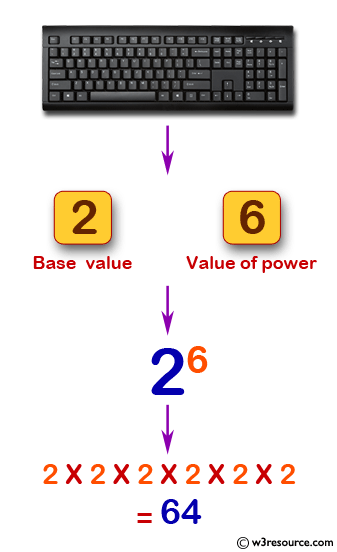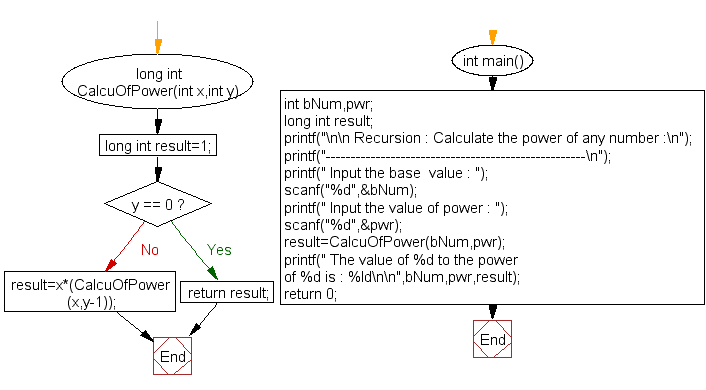﻿ C Program: Calculate the power of any number - w3resource

# C Exercises: Calculate the power of any number

## C Recursion : Exercise-17 with Solution

Write a program in C to calculate the power of any number using recursion.

Pictorial Presentation:Sample Solution:

C Code:

``````#include <stdio.h>

long int CalcuOfPower(int x,int y)
{
long int result=1;
if(y == 0) return result;
result=x*(CalcuOfPower(x,y-1));  //calling the  function CalcuOfPower itself recursively
}
int main()
{
int bNum,pwr;
long int result;
printf("\n\n Recursion : Calculate the power of any number :\n");
printf("----------------------------------------------------\n");

printf(" Input the base  value : ");
scanf("%d",&bNum);

printf(" Input the value of power : ");
scanf("%d",&pwr);

result=CalcuOfPower(bNum,pwr);//called the function CalcuOfPower

printf(" The value of %d to the power of %d is : %ld\n\n",bNum,pwr,result);

return 0;
}
```
```

Sample Output:

``` Recursion : Calculate the power of any number :
----------------------------------------------------
Input the base  value : 2
Input the value of power : 6
The value of 2 to the power of 6 is : 64
```

Explanation:

```long int CalcuOfPower(int x,int y)
{
long int result=1;
if(y == 0) return result;
result=x*(CalcuOfPower(x,y-1));  //calling the  function CalcuOfPower itself recursively
}
```

The function ‘CalcuOfPower()’ takes two integer parameters ‘x’ and ‘y’ and returns a long int result.

• A variable result is initialized to 1.
• The base case is checked where if y is 0, then the function returns result which is 1.
• If y is not 0, then the function recursively calls itself with y-1 until y becomes 0.
• In each recursive call, result is multiplied with x and assigned back to result.
• Finally, the function returns the result which is the power of x raised to y.

Time complexity and space complexity:

The time complexity of the function is O(n) - where n is the value of y, since the function is called recursively y times.

The space complexity of the function is O(n) - where n is the value of y, since n stack frames are used for the recursive function calls.

Flowchart:C Programming Code Editor:

Have another way to solve this solution? Contribute your code (and comments) through Disqus.

What is the difficulty level of this exercise?

Test your Programming skills with w3resource's quiz.

﻿

## C Programming: Tips of the Day

__FILE__ macro shows full path

```#include <string.h>

#define __FILENAME__ (strrchr(__FILE__, '/') ? strrchr(__FILE__, '/') + 1 : __FILE__)
For Windows use '\\' instead of '/'.
```

Ref : https://bit.ly/3iEWRoT

We are closing our Disqus commenting system for some maintenanace issues. You may write to us at reach[at]yahoo[dot]com or visit us at Facebook